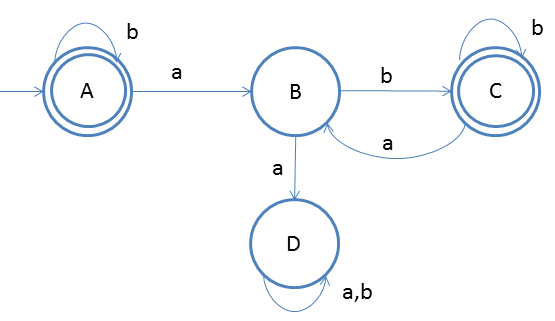# DFA String Examples

Design a DFA in which every 'a' should be followed by 'b'
Given: Input alphabet, Σ={a, b}
Language L = {ε, ab, abab, bbbb, ...}

Clearly the language is infinite because there is infinite number of strings.The idea behind this approach is very simple, follow the steps below and you will understand.

1. Firstly for ε we will make the start sate as final state.
2. If the start input comes 'a' then we should have one 'b' as the next input and we should reach final state, that is why state B and C
3. Suppose after first input 'a' again 'a' came then we should reject it, that is why we have state D and on state D we do not care for either a or b
4. Here D state is a dead state

### Testing

1. Lets take one input string abbab
2. Scan string from left to right
3. First input is a, so from state A we will go to state B
4. Second input is b, so from state B we will go to state C
5. Third input is b, so from state C we will go to state C itself
6. Fourth input is a, so from state C we will go to state B
7. Fifth input is b, so from state B we will go to state C(final state)
After end of the string we are at final state so string is accepted.
You may also try some examples of strings to run over this DFA, and you will understand more